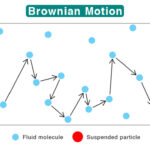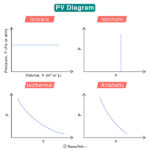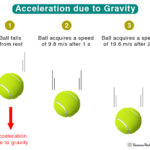Home / Physics / Faraday’s Law

Faraday’s law of electromagnetic induction states that a voltage is induced in a conductor whenever relative motion exists between the conductor and an externally applied magnetic field. The magnitude of this voltage is proportional to the rate of change of the magnetic flux. Thus, the law establishes a quantitative relationship between the changing magnetic flux and the induced voltage in an electromagnetic circuit.

### History

This law is credited to English scientist Michael Faraday, who contributed significantly to electromagnetism and electrochemistry. In 1831, Faraday demonstrated electromagnetic induction for the first time by wrapping two wires around opposite sides of an iron ring. One wire was connected to a battery that gave rise to a current, and the other wire to a galvanometer. The current in the first wire generated a magnetic field, which passed through the second wire. When the magnetic flux changed, a current was induced in the second wire as detected by the galvanometer.

Consider the example of a conducting wire wound into a coil. Suppose this coil is placed in an external magnetic field, represented by magnetic lines of forces. The number of lines passing through a given cross-sectional area is defined as the magnetic flux. There are several ways to change this flux.

According to Faraday’s law, a change in magnetic flux gives rise to an induced voltage, called electromotive force (emf). Mathematically, the law is given by the following formula.

ε = – N dφ/dt

Where,

ε = Induced emf

N = number of turns of the coil

dφ/dt = instantaneous change in magnetic flux with time

SI Unit of EMF: Volts

The negative sign is because the induced emf opposes the magnetic field. The magnitude of the emf is given by,

|ε| = N dφ/dt

When, N = 1, and dφ/dt = 1 Wb/s, then |ε| = 1 V

Therefore, the induced emf is the voltage generated across a coil of one turn when the flux rate is 1 Wb/s. Lenz’s law determines the direction of the induced current.

Scottish mathematician James Clerk Maxwell developed a set of partial differential equations that describe how electric and magnetic fields are generated by charges, currents, and changes of the fields. Today, these equations are collectively known as Maxwell’s equation. An earlier version of the equations was first published in 1861. The Maxwell-Faraday equation is given by,

X E = -∂B/∂t

Where,

B: Magnetic Field

Faraday’s law is one of the most fundamental laws of electromagnetism. This law finds its application in most electrical devices. It can explain the working principle of transformers, generators, inductors, and motors. Here are a few uses and applications, including some in daily life.

• Transformer: It consists of a pair of coils wound around a square core. Alternating current passing through one coil generates changing magnetic field, resulting in an induced current in the second coil.
• Generator: A device that converts mechanical energy into electrical energy used in an external circuit. A conductor coil is rotated rapidly between the poles of a horseshoe-type magnet. The rotating coil intercepts the magnetic field, and a current is induced in it.
• Induction cooker: It is used to heat a cooking vessel by induction instead of flame or electrical coil. It uses the concept of mutual inductance, which is based on the principle of mutual induction.
• Electrical bells: A device that functions using an electromagnet. An electromagnetically operated hammer strikes a bell resulting in sound.
• Electromagnetic Flow Meter: A device used to measure the velocity of conducting fluids by applying a magnetic field. The induced emf is proportional to the fluid velocity.

Article was last reviewed on Thursday, February 2, 2023

### Related articlesBrownian MotionPV DiagramVelocity vs AccelerationAcceleration due to Gravity